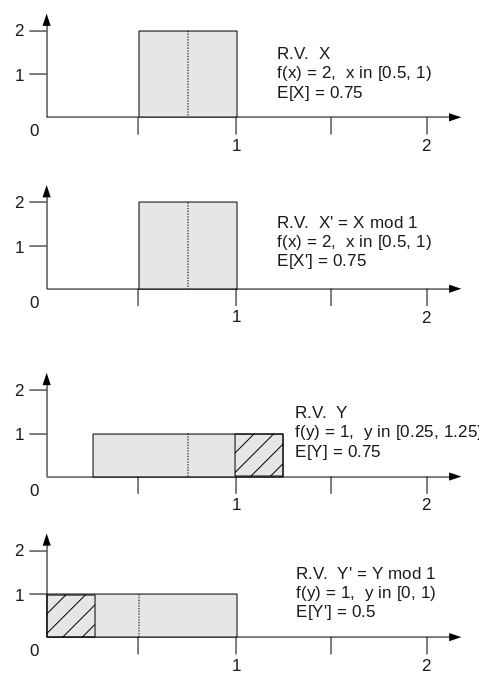I’ve been considering models for the effects of HTCondor negotiation cycle cadence on pool loading and accounting group starvation, which led me to thinking about the effects of taking the modulus of a random variable, for reasons I plan to discuss in future posts.

When you take the modulus of a random variable, X, the corresponding expected value E[X mod m] is not equal to E[X] mod m. Consider the following example:As we see from the example above, the random variables X and Y have the same mean: E[X] = E[Y] = 0.75, however E[X mod 1] = 0.75 while E[Y mod 1] = 0.5. One implication is that computing the moments of the modulus of random variables must be on a per-distribution basis, perhaps via monte carlo methods.